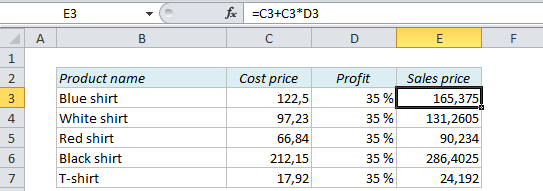# How to calculate prices and make them end with a certain number in Excel

## How to calculate prices and make them end with a certain number in Excel

When you calculate prices you usually know the cost price and the desired profit percentage, so all you have to do is to add the profit to the cost price in order to calculate the sales price. However, the price list might not look as nice as you want: